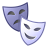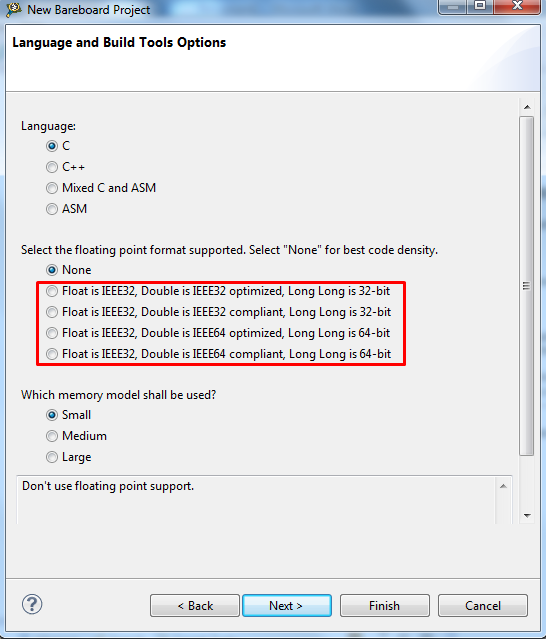キャンセル

もしかして:

# S12Z voltage measurement

## S12Z voltage measurement

The ADC measurement is always relative – relative to your voltage reference.

The most of the applications don't allow to use accurate and expensive voltage reference. In that case, VDDA is used as the reference for ADC measurement.

Since the operational VSUP range starts from 3.5V and accuracy of the voltage regulator is limited, we should use internal bandgap reference for compensating ADC voltage results. The bandgap voltage VBG has a narrow variation over temperature and external voltage supply.

The example shows how to compensate ADC results by additional bandgap voltage measurement.

ラベル(1)
• ### General

コメントI can't compile through,in the  line as follow:
//We could calculate measured voltage with fix reference voltage 5V
pot = (pot_raw * (float) REFERENCE_VOLTAGE)/4095;

can you tell me while,TKS.Hi Shen,

This line use re-type to float. I suppose that this may be a root cause.

If you want to use float type, you have to use float library.

You may create new project with float supportor, you may edit project settings and replace standard C no float library ansii.lib by for example C float library ansif.libNote:

1. Float is IEEE32, Double is IEEE32 optimized, Long Long is 32-bit

ANSI library: ansif.lib

Compiler options:

2. Float is IEEE32, Double is IEEE32 compliant, Long Long is 32-bit

ANSI library: ansifc.lib

Compiler options: -fp_compliant

3. Float is IEEE32, Double is IEEE64 optimized, Long Long is 64-bit

ANSI library: ansidm.lib

Compiler options: -double_size=8 -bit64_code_gen mem

4. Float is IEEE32, Double is IEEE64 compliant, Long Long is 64-bit

ANSI library: ansidmc.lib

Compiler options: -fp_compliant -double_size=8 -bit64_code_gen mem

I hope it helps you.

Have a great day,